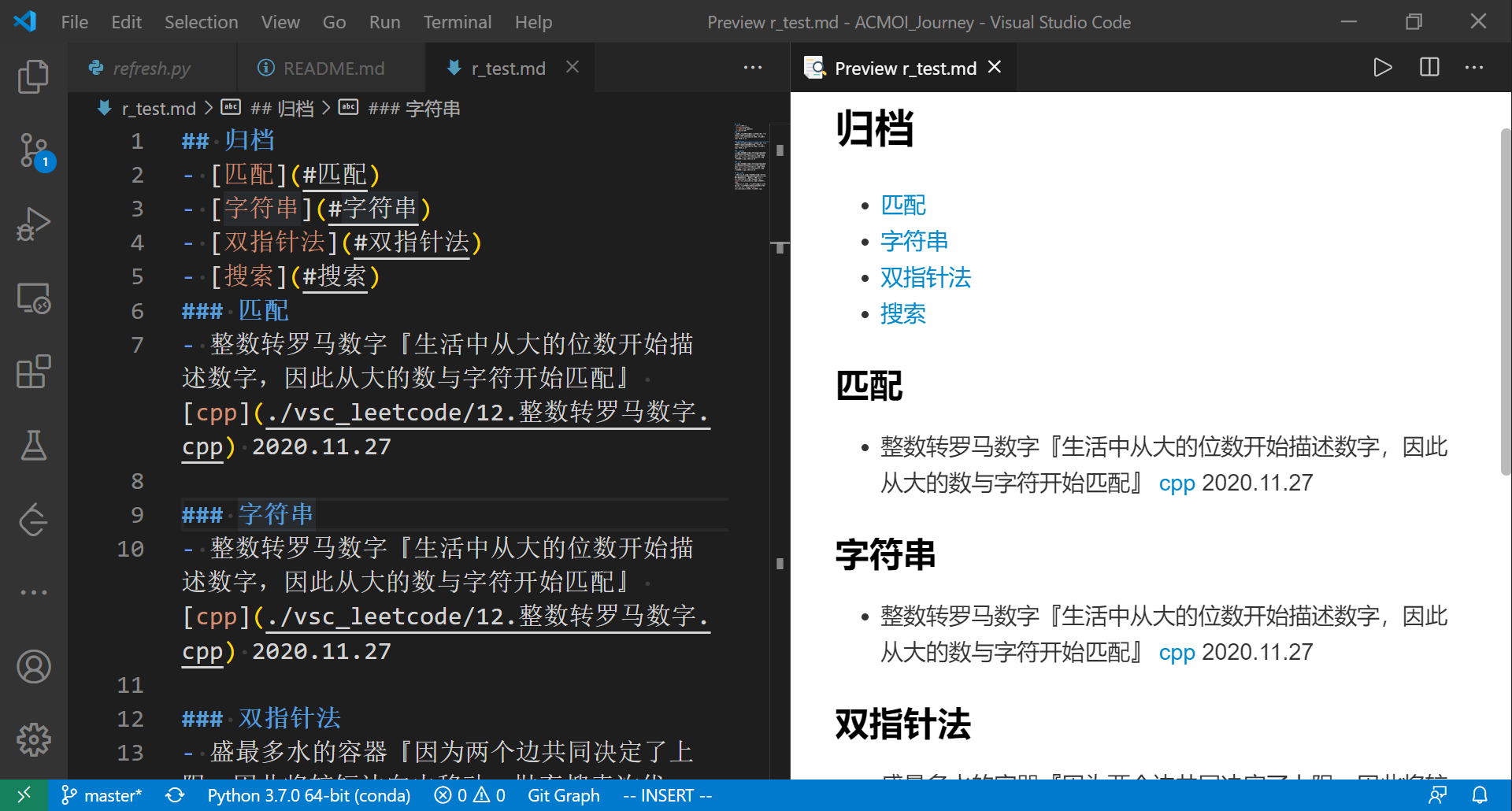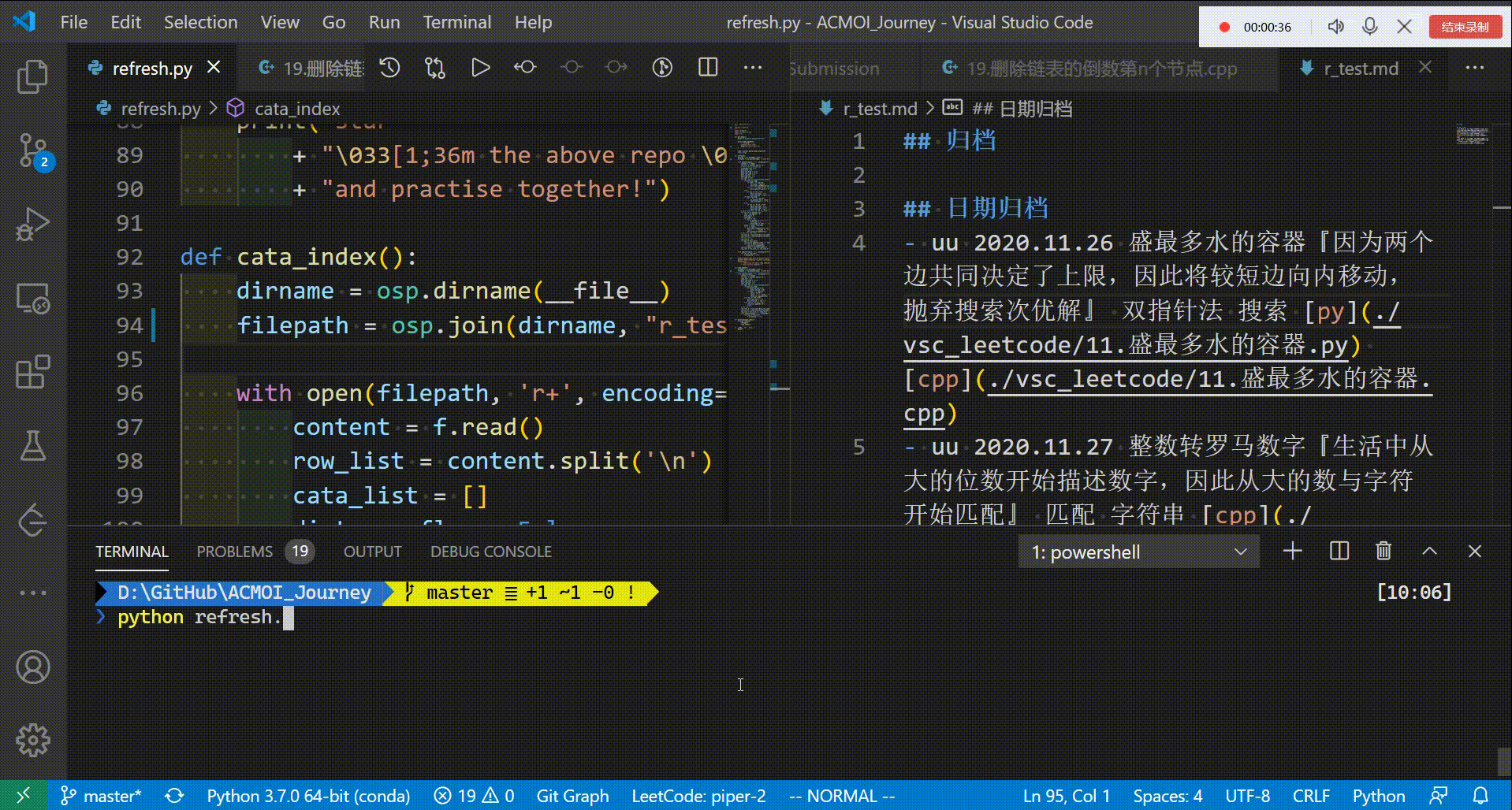## 「懒惰的美德」我用 python 写了个自动生成给文档生成索引的脚本

2020/12/5 10:45:25 文章标签:

# 我用 python 写了一个自动生成索引的脚本

### 需求实现

``````# ACM/OI Journey

- OI Wiki: https://oi-wiki.org/
- 力扣中国: https://leetcode-cn.com/

## 归档
## 日期归档
``````

``````## 日期归档
- uu 2020.11.26 盛最多水的容器『因为两个边共同决定了上限，因此将较短边向内移动，抛弃搜索次优解』 双指针法 搜索 [py](./vsc_leetcode/11.盛最多水的容器.py) [cpp](./vsc_leetcode/11.盛最多水的容器.cpp)
- uu 2020.11.27 整数转罗马数字『生活中从大的位数开始描述数字，因此从大的数与字符开始匹配』 匹配 字符串 [cpp](./vsc_leetcode/12.整数转罗马数字.cpp)
``````

``````## 归档
- [匹配](#匹配)
- [字符串](#字符串)
- [双指针法](#双指针法)
- [搜索](#搜索)
### 匹配
- 整数转罗马数字『生活中从大的位数开始描述数字，因此从大的数与字符开始匹配』 [cpp](./vsc_leetcode/12.整数转罗马数字.cpp) 2020.11.27

### 字符串
- 整数转罗马数字『生活中从大的位数开始描述数字，因此从大的数与字符开始匹配』 [cpp](./vsc_leetcode/12.整数转罗马数字.cpp) 2020.11.27

### 双指针法
- 盛最多水的容器『因为两个边共同决定了上限，因此将较短边向内移动，抛弃搜索次优解』 [py](./vsc_leetcode/11.盛最多水的容器.py) [cpp](./vsc_leetcode/11.盛最多水的容器.cpp) 2020.11.26

### 搜索
- 盛最多水的容器『因为两个边共同决定了上限，因此将较短边向内移动，抛弃搜索次优解』 [py](./vsc_leetcode/11.盛最多水的容器.py) [cpp](./vsc_leetcode/11.盛最多水的容器.cpp) 2020.11.26

## 日期归档
- 2020.11.26 盛最多水的容器『因为两个边共同决定了上限，因此将较短边向内移动，抛弃搜索次优解』 双指针法 搜索 [py](./vsc_leetcode/11.盛最多水的容器.py) [cpp](./vsc_leetcode/11.盛最多水的容器.cpp)
- 2020.11.27 整数转罗马数字『生活中从大的位数开始描述数字，因此从大的数与字符开始匹配』 匹配 字符串 [cpp](./vsc_leetcode/12.整数转罗马数字.cpp)
``````### 整体逻辑

• 先把文件读到内存中，以列表`list`的形式保存
• 列表`list`内，每一元素对应一句话
• 遍历列表，遇到元素`## 归档`则其之后的元素按照不同条件取出、分析
• 直到遇到元素`## 日期归档`，则把其之后的元素按条件取出、分析

``````""" """
import os.path as osp
import re
def refreah():
"""
那么我获取其绝对路径
注意这里处理的文件和代码文件处于同一目录下
"""
dirname = osp.dirname(__file__)

"""
打开这个文件，其变量名是 f
"""
with open(filepath, 'r+', encoding='utf-8') as f:
"""
"""
"""
以“换行符”/“回车”进行字符串分割
这样，row_list 每个元素就是一行文字了
"""
row_list = content.split('\n')
"""
下面开始把不同的目录对应的条目取出
"""
# found the un-packed row
un_packed_rows = []
dict_cata = {}
dict_row_flag = False
date_row_flag = False
dict_row_num  = 0
date_row_num  = 0
cur_cata = None
for idx, row in enumerate(row_list):
"""
如果到了 ## 归档 下面
"""
if dict_row_flag:
if "### " in row[:4]:
cur_cata = row[4:]
"""
data_cata 是我们的类别字典，最终效果为
data_cata = {
"匹配": [匹配的第1题, 匹配的第2题, ...],
"字符串": [字符串的第1题, 字符串的第2题, ...],
...
}
"""
dict_cata.setdefault(cur_cata, [])
elif "- " in row[:2] and not re.match('\[.*\]\(.*\)', row[2:]):
"""
这里用了一个正则
因为索引格式为
- [索引名称](#索引名称)
而题目格式为
- 题目 程序 日期
因此如果仅凭是否以「- 」开头，则难以区分二者
因此加了一个是否正则匹配 [*](*) 的判断
"""
dict_cata[cur_cata] = [row] + dict_cata[cur_cata]
else:
"""
判断是否到了 ## 归档 下面
"""
if row == "## 归档":
dict_row_flag = True
dict_row_num  = idx + 1
"""
如果到了 ## 日期归档 下面
"""
if date_row_flag:
"""
- uu 是我自己设的格式
如果题目有 uu ，那么这条就是我要用脚本加到归档里的题目
"""
if '- uu ' in row[:5]:
un_packed_rows = [row] + un_packed_rows
row_list[idx] = "- " + row[5:]
else:
"""
判断是否到了 ## 日期归档 下面
"""
if row == "## 日期归档":
date_row_flag = True
dict_row_flag = False
date_row_num  = idx + 1
# pack those rows to "## 日期归档"
"""
下面是把新题目（uu）加到 data_cata 字典中
"""
for row in un_packed_rows:
row = row.split(' ')
file_num = 0
file_name = ""
for ele in row:
if re.match('\[.*\]\(.*\)', ele):
file_num += 1
file_name += (ele + ' ')
catas = row[4:-file_num]
for c in catas:
dict_cata.setdefault(c, [])
row_ = '- ' + row + ' ' + file_name + row
dict_cata[c].append(row_)
# del file "## 归档"
"""
下面是清空 ## 归档 的内容
根据 dict_cata 书写新的全部内容
"""
row_list_a = row_list[:dict_row_num]
row_list_c = row_list[date_row_num-2:]
## row_list_b
row_list_b = []
for key in dict_cata:
row_list_b.append("\n### " + key)
for row in dict_cata[key]:
row_list_b.append(row)
row_list_b = row_list_b[1:]
row_list = row_list_a + row_list_b + row_list_c

"""
把新处理好的文本，逐行写到文件中
（文件先清空，原文本被覆盖）
"""
with open(filepath, 'w', encoding='utf-8') as f:
for row in row_list:
f.write(row + '\n')

"""
提示用户，处理好了
"""
print("\033[1;36mhttps://github.com/PiperLiu/ACMOI_Journey\033[0m")
print("star"
+ "\033[1;36m the above repo \033[0m"
+ "and practise together!")

def cata_index():
"""
这是我用于生成索引的函数
索引就是：
## 归档
- [匹配](#匹配)
- [字符串](#字符串)
- [双指针法](#双指针法)
- [搜索](#搜索)

思路很简单，还是取各个三级标题
然后规整到 ## 归档 下面
"""
dirname = osp.dirname(__file__)

with open(filepath, 'r+', encoding='utf-8') as f:
row_list = content.split('\n')
cata_list = []
dict_row_flag = False
dict_row_num  = 0
cata_row_num  = 0
for idx, row in enumerate(row_list):
if dict_row_flag:
if cata_row_num == 0:
cata_row_num = idx
if "### " in row[:4]:
cata = row[4:]
cata = "- [" + cata + "]" + "(#" + cata + ")"
cata_list.append(cata)
elif row == "## 归档":
dict_row_flag = True
dict_row_num  = idx + 1
elif row == "## 日期归档":
cata_list.append("\n")
break
row_list_a = row_list[:dict_row_num]
row_list_c = row_list[cata_row_num:]
row_list = row_list_a + cata_list + row_list_c
with open(filepath, 'w', encoding='utf-8') as f:
for row in row_list:
f.write(row + '\n')

refresh()
cata_index()
``````### argparse应用

``````def get_args():
parser = argparse.ArgumentParser()

'--refresh', '-r',
action='store_true',
)

args = parser.parse_known_args()
return args
``````

``````def main(args=get_args()):
if args.refresh:
refreah()
cata_index()

if __name__ == "__main__":
main()
``````

### 祝各位变得更强。欢迎关注公众号：`Piper蛋窝`，回复`微信`加我微信，邀请你进入高质量技术交流群 / 好文分享群。欢迎`点赞`、点击`在看`将好文分享出去。暂无相关的数据...